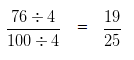# Convert Percent to Fraction | Maths Grade 7

### Convert Percent to Fraction

Step 1 – In order to convert Percent to Fraction, we divide the Percentage by 100, and remove the sign of Percentage (%).

Step 2 – Simplify the Fraction

### Convert Percent to Fraction Examples

Example 1

Convert 50 % into a Fraction:

Explanation

50 % = 50/100

On Simplifying, 50/100

HCF of 50 and 100 is 50

Divide both 50 and 100 by their HCF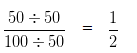Example 2

Convert 40 % into a Fraction:

Explanation

40 % = 40/100

On Simplifying, 40/100

HCF of 40 and 100 is 20

Divide both 40 and 100 by their HCF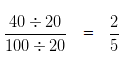Example 3

Convert 65 % into a Fraction:

Explanation

65 % = 65/100

On Simplifying, 65/100

HCF of 65 and 100 is 5

Divide both 65 and 100 by their HCF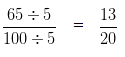Example 4

Convert 80 % into a Fraction:

Explanation

80 % = 80/100

On Simplifying, 80/100

HCF of 80 and 100 is 20

Divide both 80 and 100 by their HCF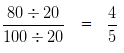Example 5

Convert 75 % into a Fraction:

Explanation

75 % = 75/100

On Simplifying, 75/100

HCF of 75 and 100 is 25

Divide both 75 and 100 by their HCF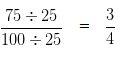Example 6

Convert 88 % into a Fraction:

Explanation

88 % = 88/100

On Simplifying, 88/100

HCF of 88 and 100 is 4

Divide both 88 and 100 by their HCF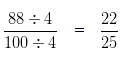Example 7

Convert 90 % into a Fraction:

Explanation

90 % = 90/100

On Simplifying, 90/100

HCF of 90 and 100 is 10

Divide both 90 and 100 by their HCF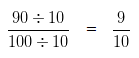Example 8

Convert 76 % into a Fraction:

Explanation

76 % = 76/100

On Simplifying, 76/100

HCF of 76 and 100 is 4

Divide both 76 and 100 by their HCF